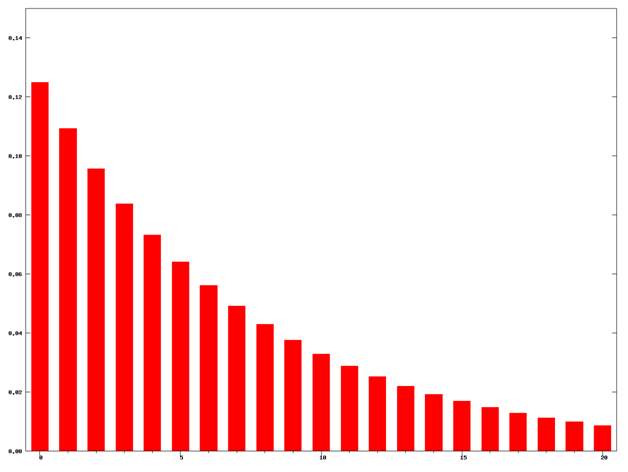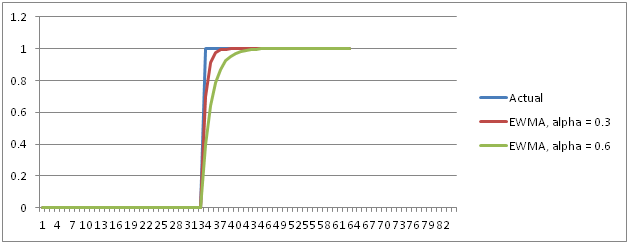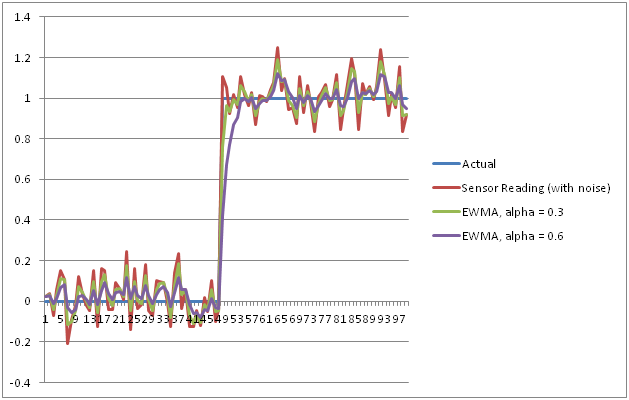# Filtering Noise from Sensor Readings: A Simple Low Pass Filter

In a perfect world, a sensor’s output would exactly match the input it is sensing.  In the real world, there are a number of errors introduced, including bias, time lags in response, and noise.  This article discusses one of the simplest filters for reducing noise: the Exponentially Weighted Moving Average (EWMA) filter.  Despite its long name, it’s about the simplest and most efficient filter you can implement.  The name comes from the concept that the filter’s output, y, is the weighted average of the current input and each previous inputs, with the weighting decreasing exponentially:

Yt = α (Xt + (1 – α) Xt-1 + (1-α)2 Xt-2 + (1-α)3 Xt-3 + ….

where Yt is the current output from the filter, and Xt is the current input, and the other X’s are each preceding value.  The weighting is shown in the figure:This averaging tends to smooth out the response and reduce noise.  Of course, this formula would be cumbersome and time consuming to implement in an embedded system.  Even if only a small number of past values were used.  Some math can show that the formula can be rewritten as a recursive formula using the past output from the filter:

Yt = α Yt-1  + (1 – α) Xt for all values of t > 0, or, re-arranging terms:

Yt =  Xt  + α ( Yt-1 – Xt)

This means that all you need to store is the immediate past output of the filter, which already has the weighted average of all previous values calculated in. This is simpler and takes less storage than, for example, averaging the current and past 4 values of the sensor.  It will also respond more quickly to actual changes that you are trying to measure.

## Limits

You can choose a value for α ranging between 0 and 1.  At 0, the output is just the raw input: no filtering occurs.  The output will respond instantly both to noise and to true changes in the system.

At 1, the output becomes equal to the past value: it never changes, eliminating all noise and all real signal changes!  Clearly one wants to select a value in between these extremes.

## Selecting α

The correct value will depend on your situation.  The higher the a, the more noise if filtered out, but also the more lag that is introduced in reacting to real system changes.  This is shown in the following figures:This figure shows the response of two filters to a unit step function.  The red curve is for α = 0.3, and the green curve is forα = 0.6.This graph shows the output of the filters to a noisy sensor, with Gaussian noise with a standard deviation of 0.1.  One can see from the graph that a value of 0.3 reduces the noise somewhat, but leaves a lot in.  A value of 0.6 greatly reduces the noise, at the expense of greater lag.

## Initializing the Filter

The formulas work for all values after the initial run of the filter.  Like any recursive formula there needs to be a starting value.  My rather rough and informal scanning of the literature identified three common methods:

• For the first pass, set the output equal to the input (same as no filtering)
• Take a small number of readings, e.g.,  5, and use a straight average of these as the initial output.   This is what I did for compass readings in my current robotic vehicle.
• For control processes, an alternative is to set the initial output at the desired control point (figuring, I suppose, that if the control system is working, the average should be at the control point).

## Summary

The simple EWMA routing can be summarized as:

For t= 0:

Yt = Xt

Else:

Yt =  Xt  + α ( Yt-1 – Xt)

There are many different types of filters, each with advantages and disadvantages. No one filter is best for all applications.  I’m just a hobbyist, and don’t pretend to have much knowledge in this area, but there is a wealth of information out there.

## 3 thoughts on “Filtering Noise from Sensor Readings: A Simple Low Pass Filter”

1. Hi Mike!

2.cesare on said: